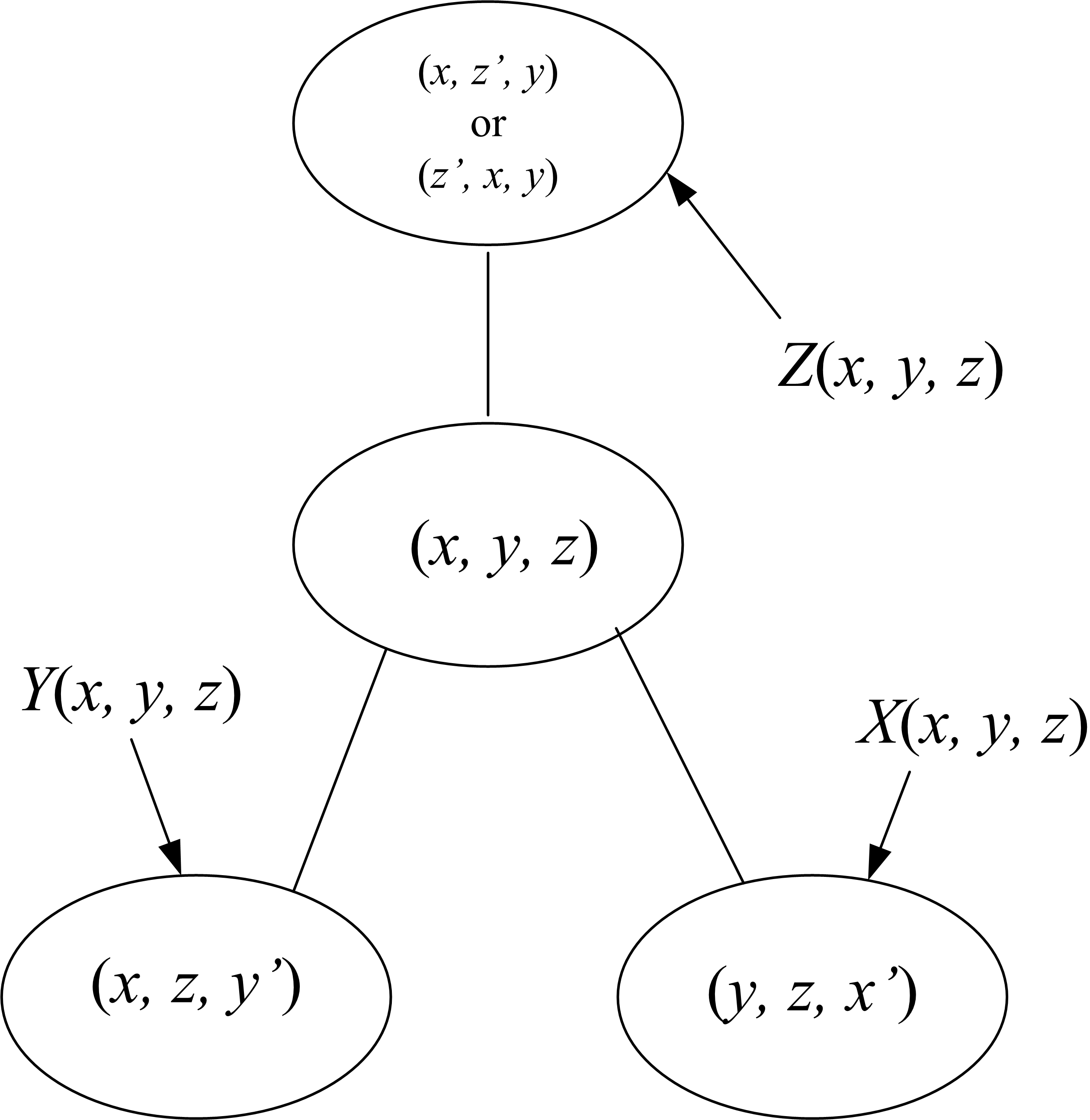# Markov numbers applet

## General properties

This page displays an applet showing the tree of Markov numbers. It gives the basic description of the tree and the instructions for navigating in that tree. To display the tree, click on the bluish button, labeled "Display the Markov Tree". Depending on your security setting, a yellow bar at the top of the page may appear, warning you that running this applet ("ActiveX controls" it will say) maybe dangerous. In that case, click on this yellow bar and allow the applet to be run. Trust me. In any case, after you click on the bluish button, the tree will appear in a separate window. You may resize the window, minimize it (in which case its icon will appear in the bottom tray so you can restore it), or close it altogether. If this last action is taken, you must click on the bluish button again to restore the applet.

The Markov numbers are the solutions of the diophantic equation:(1)

Each node in the tree holds a solution triplet (x, y, z) with x ≤ y ≤ z. The two children of a node are generated as follows: Suppose

(x, y, z),      x ≤ y ≤ z

is a solution held at some node. If we hold two of the variables fixed, this defines a quadratic equation in the third variable. That equation is monic, with integer coefficients, thus the other root, denoted by x', y', or z', is also an integer, giving rise to another solution. This produces three other triplets solving the equation (1):

(y, z, x') denoted by X(x, y, z)

(x, z, y') denoted by Y(x, y, z) and

(z', x, y) or (x, z', y) denoted by Z(x, y, z)

The smallest solution, strictly speaking, is (1, 1, 1), which gives rise to three identical triplets (1, 1, 2). That triple, in turn, produces two triplets (1, 2, 5), and one already accounted for: (1, 1, 1). The triplets (1, 1, 1) and (1, 1, 2) are the only solutions in which x = y or y = z. Every other node (x, y, z) in the tree has then two new distinct children: The left child: (x, z, y') and the right child: (y, z, x'), with y' < x' . The triplet containing z', be it (z', x, y) or (x, z', y), already appears further up the tree, in fact it is the parent of (x, y, z). The reason for this is that if x < y < z then:

z < x',   z < y',    y' < x' , and     z' < y

Thus, if we display the tree with (1, 2, 5) at the root, the tree is quite regular, every node has two children as described above. The tree structure at the node (x, y, z) can be described by this picture showing the location of the nodes X(x, y, z), Y(x, y, z) and Z(x, y, z):

Parent Z(x, y, z) = (x, z', y) or (z', x, y)Left child Y(x, y, z) = (x, z, y')
Right child X(x, y, z) = (y, z, x')

See the mathematical discussion for all the proofs and further references.

It is a long standing conjecture, going back to Frobenius in 1913, whether the tree, as described, defines each Markov number uniquely. More precisely, given a Markov number m, is there only one triplet of the form (x, y, m)? (With x ˂ y ˂ m, of course.)